## [MCQ’s] Theory of Computer Science

### Theory of Computer Science

1. Assume the R is a relation on a set A, aRb is partially ordered such that a and b are _____________
a) reflexive
b) transitive
c) symmetric
d) reflexive and transitive

Explanation: A partially ordered relation refers to one which is Reflexive, Transitive and Antisymmetric.

2. The non- Kleene Star operation accepts the following string of finite length over set A = {0,1} | where string s contains even number of 0 and 1
a) 01,0011,010101
b) 0011,11001100
c) ε,0011,11001100
d) ε,0011,11001100

Explanation: The Kleene star of A, denoted by A*, is the set of all strings obtained by concatenating zero or more strings from A.

3. A regular language over an alphabet ∑ is one that cannot be obtained from the basic languages using the operation
a) Union
b) Concatenation
c) Kleene*
d) All of the mentioned

Explanation: Union, Intersection, Concatenation, Kleene*, Reverse are all the closure properties of Regular Language.

4. Statement 1: A Finite automata can be represented graphically; Statement 2: The nodes can be its states; Statement 3: The edges or arcs can be used for transitions
Hint: Nodes and Edges are for trees and forests too.
Which of the following make the correct combination?
a) Statement 1 is false but Statement 2 and 3 are correct
b) Statement 1 and 2 are correct while 3 is wrong
c) None of the mentioned statements are correct
d) All of the mentioned

Explanation: It is possible to represent a finite automaton graphically, with nodes for states, and arcs for transitions.

5. The minimum number of states required to recognize an octal number divisible by 3 are/is
a) 1
b) 3
c) 5
d) 7

Explanation: According to the question, minimum of 3 states are required to recognize an octal number divisible by 3.

6. Which of the following is a not a part of 5-tuple finite automata?
a) Input alphabet
b) Transition function
c) Initial State
d) Output Alphabet

Explanation: A FA can be represented as FA= (Q, ∑, δ, q0, F) where Q=Finite Set of States, ∑=Finite Input Alphabet, δ=Transition Function, q0=Initial State, F=Final/Acceptance State).

7. If an Infinite language is passed to Machine M, the subsidiary which gives a finite solution to the infinite input tape is ______________
a) Compiler
b) Interpreter
d) None of the mentioned

Explanation: A Compiler is used to give a finite solution to an infinite phenomenon. Example of an infinite phenomenon is Language C, etc.

8. The number of elements in the set for the Language L={xϵ(∑r) *|length if x is at most 2} and ∑={0,1} is_________
a) 7
b) 6
c) 8
d) 5

Explanation: ∑r= {1,0} and a Kleene* operation would lead to the following set=COUNT{ε,0,1,00,11,01,10} =7.

9. For the following change of state in FA, which of the following codes is an incorrect option?
a) δ (m, 1) =n
b) δ (0, n) =m
c) δ (m,0) =ε
d) s: accept = false; cin >> char;
if char = “0” goto n;

Explanation: δ(QX∑) = Q1 is the correct representation of change of state. Here, δ is called the Transition function.

10. Given: ∑= {a, b}
L= {xϵ∑*|x is a string combination}
∑4 represents which among the following?

a) {aa, ab, ba, bb}
b) {aaaa, abab, ε, abaa, aabb}
c) {aaa, aab, aba, bbb}
d) All of the mentioned

Explanation: ∑* represents any combination of the given set while ∑x represents the set of combinations with length x where x ϵ I.
Crack Job Placement Aptitude in First Attempt

11. Which of the following not an example Bounded Information?
a) fan switch outputs {on, off}
c) colour of the traffic light at the moment
d) none of the mentioned

Explanation: Bounded information refers to one whose output is limited and it cannot be said what were the recorded outputs previously until memorized.

12. A Language for which no DFA exist is a________
a) Regular Language
b) Non-Regular Language
c) May be Regular
d) Cannot be said

Explanation: A language for which there is no existence of a deterministic finite automata is always Non Regular and methods like Pumping Lemma can be used to prove the same.

13. A DFA cannot be represented in the following format
a) Transition graph
b) Transition Table
c) C code
d) None of the mentioned

Explanation: A DFA can be represented in the following formats: Transition Graph, Transition Table, Transition tree/forest/Any programming Language.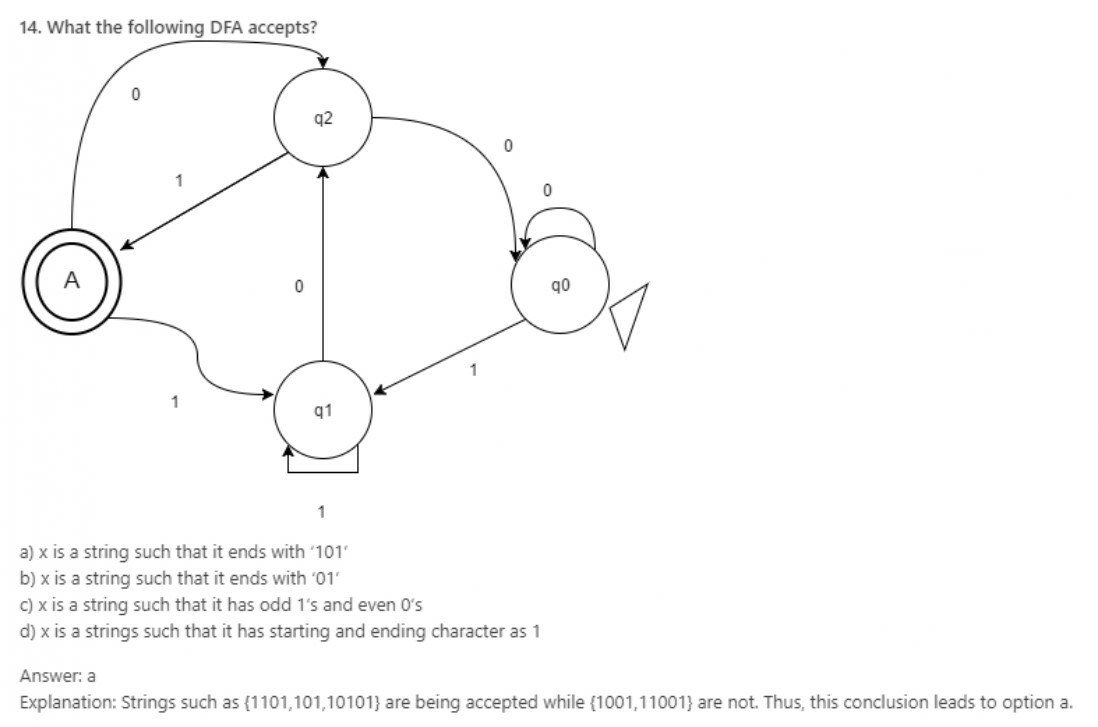15. When are 2 finite states equivalent?
a) Same number of transitions
b) Same number of states
c) Same number of states as well as transitions
d) Both are final states

Explanation: Two states are said to be equivalent if and only if they have same number of states as well as transitions.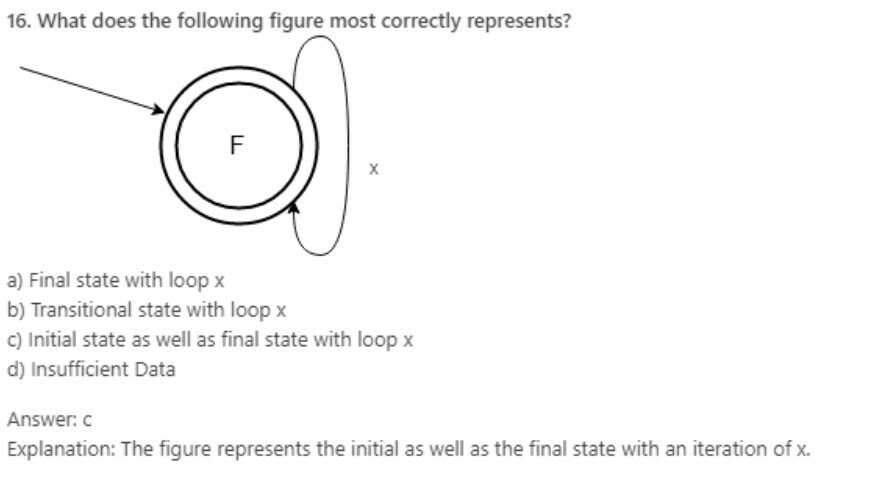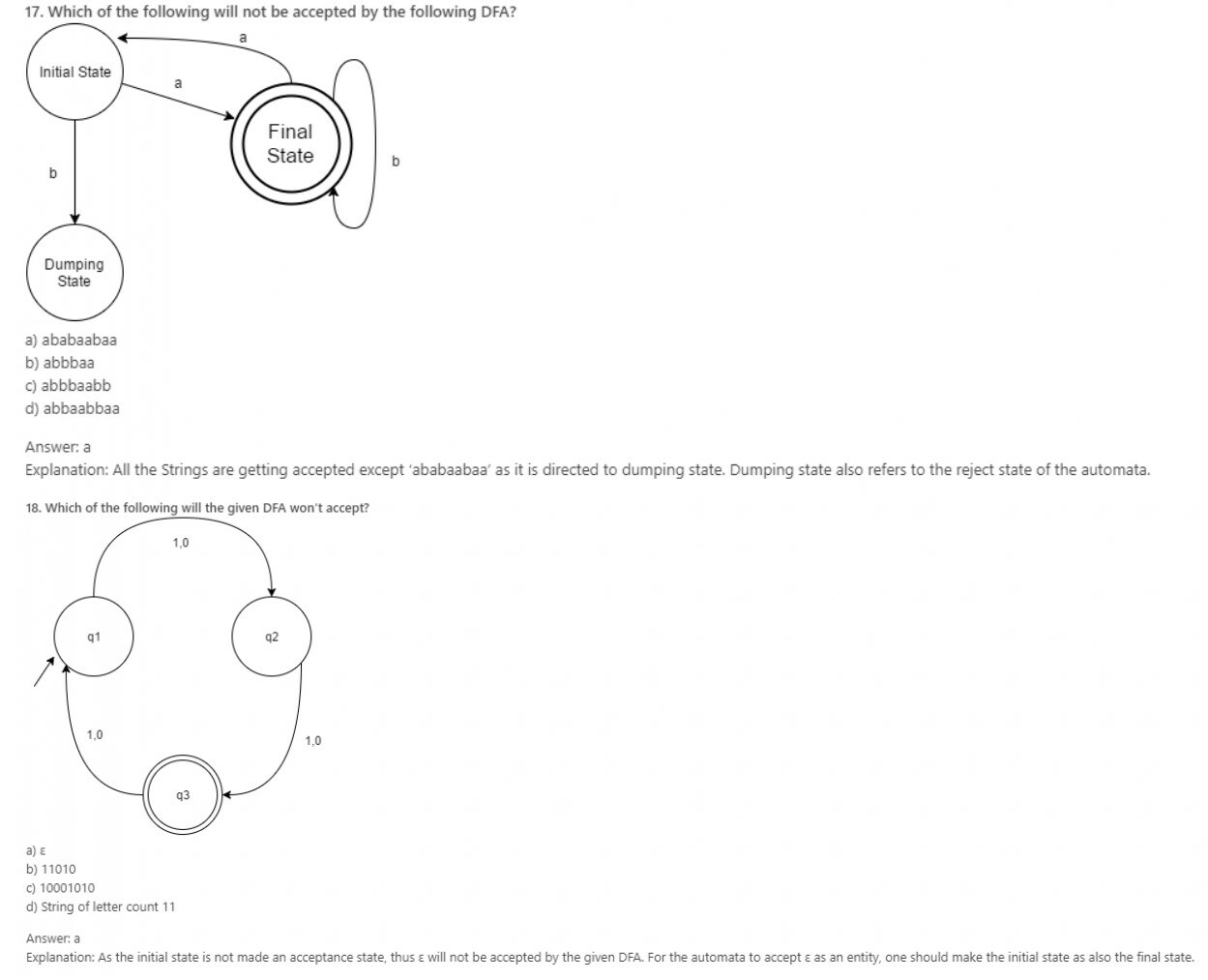19. Can a DFA recognize a palindrome number?
a) Yes
b) No
c) Yes, with input alphabet as ∑*
d) Can’t be determined

Explanation: Language to accept a palindrome number or string will be non-regular and thus, its DFA cannot be obtained. Though, PDA is possible.

20. Which of the following is not an example of finite state machine system?
a) Control Mechanism of an elevator
b) Combinational Locks
c) Traffic Lights
d) Digital Watches

Explanation: Proper and sequential combination of events leads the machines to work in hand which includes The elevator, Combinational Locks, Traffic Lights, vending machine, etc. Other applications of Finite machine state system are Communication Protocol Design, Artificial Intelligence Research, A Turnstile, etc.

Learn Machine Learning with Python from Scratch

21. Which of the following options is correct?
Statement 1: Initial State of NFA is Initial State of DFA.
Statement 2: The final state of DFA will be every combination of final state of NFA.
a) Statement 1 is true and Statement 2 is true
b) Statement 1 is true and Statement 2 is false
c) Statement 1 can be true and Statement 2 is true
d) Statement 1 is false and Statement 2 is also false

Explanation: Statement 1 and 2 always true for a given Language.

22. Given Language: L= {ab U aba}*
If X is the minimum number of states for a DFA and Y is the number of states to construct the NFA,
|X-Y|=?
a) 2
b) 3
c) 4
d) 1

Explanation: Construct the DFA and NFA individually, and the attain the difference of states.

23. An automaton that presents output based on previous state or current input:
a) Acceptor
b) Classifier
c) Transducer
d) None of the mentioned.

Explanation: A transducer is an automaton that produces an output on the basis of what input has been given currently or previous state.

24. If NFA of 6 states excluding the initial state is converted into DFA, maximum possible number of states for the DFA is ?
a) 64
b) 32
c) 128
d) 127

Explanation: The maximum number of sets for DFA converted from NFA would be not greater than 2n.

25. NFA, in its name has ’non-deterministic’ because of :
a) The result is undetermined
b) The choice of path is non-deterministic
c) The state to be transited next is non-deterministic
d) All of the mentioned

Explanation: Non deterministic or deterministic depends upon the definite path defined for the transition from one state to another or undefined(multiple paths).

26. Which of the following is correct proposition?
Statement 1: Non determinism is a generalization of Determinism.
Statement 2: Every DFA is automatically an NFA

a) Statement 1 is correct because Statement 2 is correct
b) Statement 2 is correct because Statement 2 is correct
c) Statement 2 is false and Statement 1 is false
d) Statement 1 is false because Statement 2 is false

Explanation: DFA is a specific case of NFA.

27. Given Language L= {xϵ {a, b}*|x contains aba as its substring}
Find the difference of transitions made in constructing a DFA and an equivalent NFA?
a) 2
b) 3
c) 4
d) Cannot be determined.

Explanation: The individual Transition graphs can be made and the difference of transitions can be determined.

28. The construction time for DFA from an equivalent NFA (m number of node)is:
a) O(m2)
b) O(2m)
c) O(m)
d) O(log m)

Explanation: From the coded NFA-DFA conversion.

29. If n is the length of Input string and m is the number of nodes, the running time of DFA is x that of NFA.Find x?
a) 1/m2
b) 2m
c) 1/m
d) log m

Explanation: Running time of DFA: O(n) and Running time of NFA =O(m2n).

30. Which of the following option is correct?
a) NFA is slower to process and its representation uses more memory than DFA
b) DFA is faster to process and its representation uses less memory than NFA
c) NFA is slower to process and its representation uses less memory than DFA
d) DFA is slower to process and its representation uses less memory than NFA
Explanation: NFA, while computing strings, take parallel paths, make different copies of input and goes along different paths in order to search for the result. This creates the difference in processing speed of DFA and NFA.

Crack Job Placement Aptitude in First Attempt

31. Moore Machine is an application of:
a) Finite automata without input
b) Finite automata with output
c) Non- Finite automata with output
d) None of the mentioned

Explanation: Finite automaton with an output is categorize din two parts: Moore M/C and Mealy M/C.

32. In Moore machine, output is produced over the change of:
a) transitions
b) states
c) Both
d) None of the mentioned

Explanation: Moore machine produces an output over the change of transition states while mealy machine does it so for transitions itself.

33. For a give Moore Machine, Given Input=’101010’, thus the output would be of length:
a) |Input|+1
b) |Input|
c) |Input-1|
d) Cannot be predicted

Explanation: Initial state, from which the operations begin is also initialized with a value.

34. Statement 1: Null string is accepted in Moore Machine.
Statement 2: There are more than 5-Tuples in the definition of Moore Machine.

Choose the correct option:
a) Statement 1 is true and Statement 2 is true
b) Statement 1 is true while Statement 2 is false
c) Statement 1 is false while Statement 2 is true
d) Statement 1 and Statement 2, both are false

Explanation: Even ε, when passed as an input to Moore machine produces an output.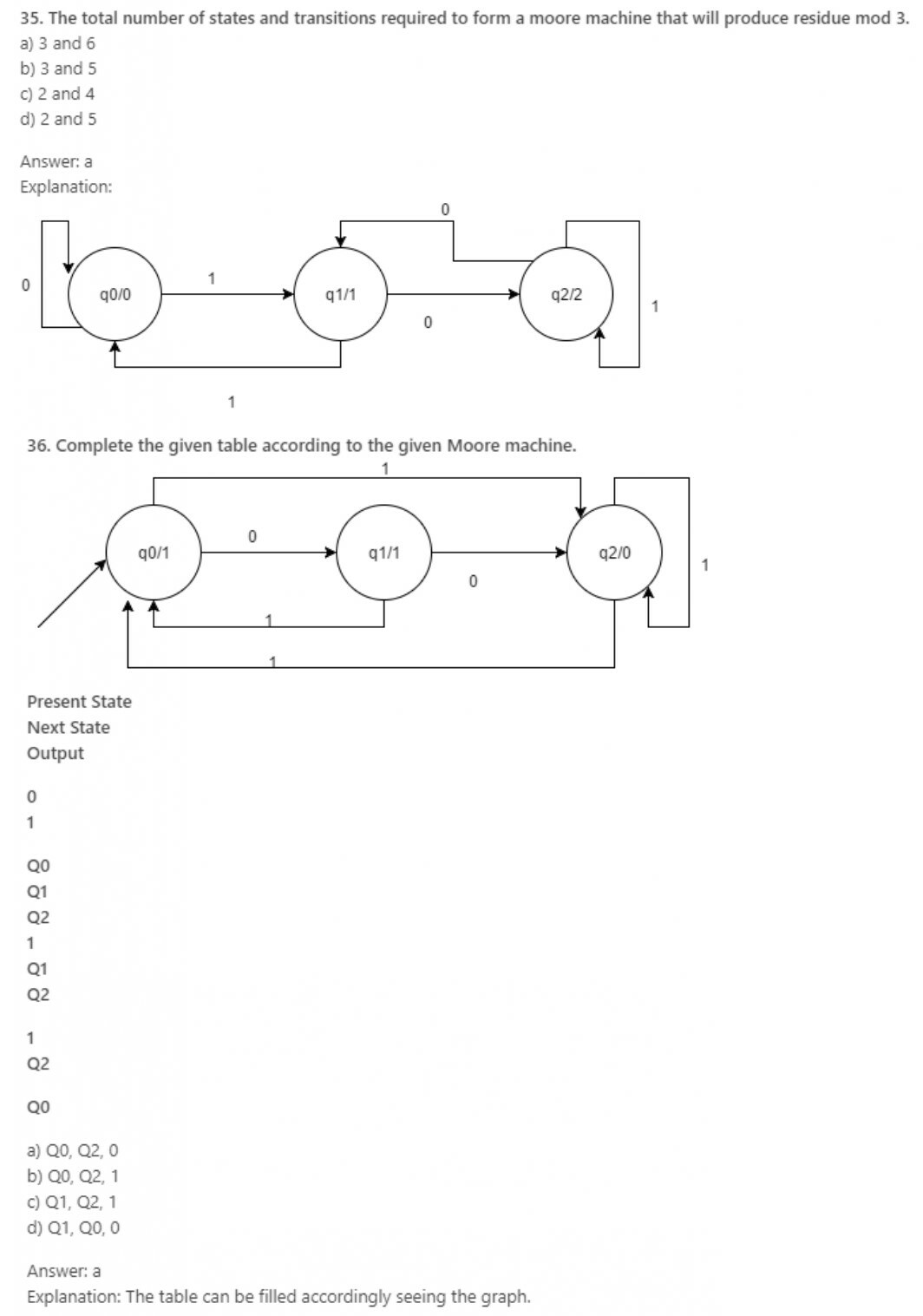37. What is the output for the given language?
Language: A set of strings over ∑= {a, b} is taken as input and it prints 1 as an output “for every occurrence of a, b as its substring. (INPUT: abaaab)
a) 0010001
b) 0101010
c) 0111010
d) 0010000

Explanation: The outputs are as per the input, produced.

38. The output alphabet can be represented as:
a) δ
b) ∆
c) ∑
d) None of the mentioned

Explanation: Source-The tuple definition of Moore and mealy machine comprises one new member i.e. output alphabet as these are finite machines with output.

39. The O/P of Moore machine can be represented in the following format:
a) Op(t)=δ(Op(t))
b) Op(t)=δ(Op(t)i(t))
c) Op(t): ∑
d) None of the mentioned

Explanation: Op(t)=δ(Op(t)) is the defined definition of how the output is received on giving a specific input to Moore machine.

40. Which of the following is a correct statement?
a) Moore machine has no accepting states
b) Mealy machine has accepting states
c) We can convert Mealy to Moore but not vice versa
d) All of the mentioned

Explanation: Statement a and b is correct while c is false. Finite machines with output have no accepting states and can be converted within each other.

Python Programming for Complete Beginners

41. In mealy machine, the O/P depends upon?
a) State
b) Previous State
c) State and Input
d) Only Input

Explanation: Definition of Mealy Machine.

42. Which of the given are correct?
a) Moore machine has 6-tuples
b) Mealy machine has 6-tuples
c) Both Mealy and Moore has 6-tuples
d) None of the mentioned

Explanation: Finite Automaton with Output has a common definition for both the categories.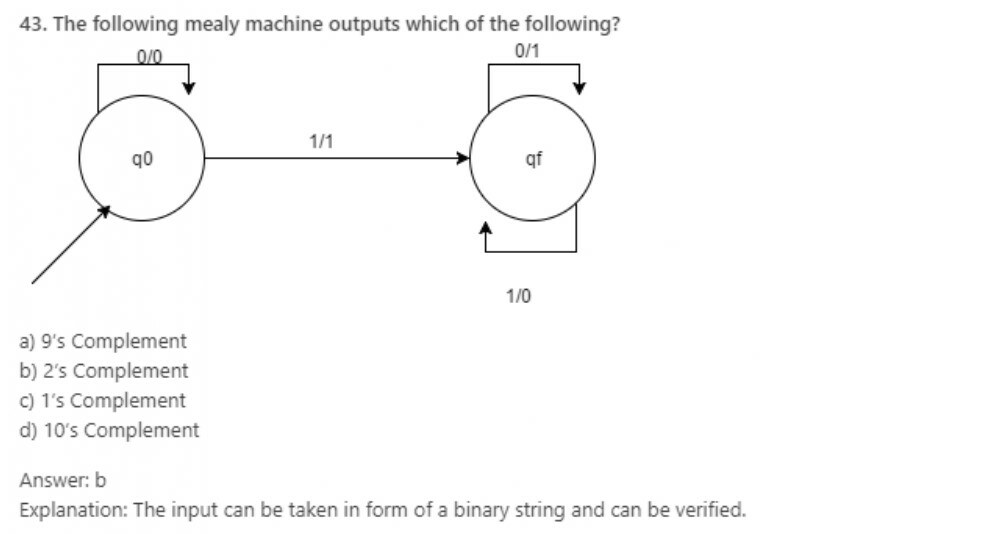44. The O/P of Mealy machine can be represented in the following format:
a) Op(t)= δ(Op(t))
b) Op(t)= δ(Op(t)i(t))
c) Op(t): ∑
d) None of the mentioned

Explanation: The output of mealy machine depends on the present state as well as the input to that state.

45.The ratio of number of input to the number of output in a mealy machine can be given as:
a) 1
b) n: n+1
c) n+1: n
d) None of the mentioned

Explanation: The number of output here follows the transitions in place of states as in Moore machine.

46. Mealy and Moore machine can be categorized as:
a) Inducers
b) Transducers
c) Turing Machines
d) Linearly Bounder Automata

Explanation: They are collectively known as Transducers.

47. The major difference between Mealy and Moore machine is about:
a) Output Variations
b) Input Variations
c) Both
d) None of the mentioned

Explanation: Mealy and Moore machine vary over how the outputs depends on prior one (transitions) and on the latter one(states).

48. Statement 1: Mealy machine reacts faster to inputs.
Statement 2: Moore machine has more circuit delays.
Choose the correct option:
a) Statement 1 is true and Statement 2 is true
b) Statement 1 is true but Statement 2 is false
c) Statement 1 is false and Statement 2 is true
d) None of the mentioned is true

Explanation: Being an input dependent and output capable FSM, Mealy machine reacts faster to inputs.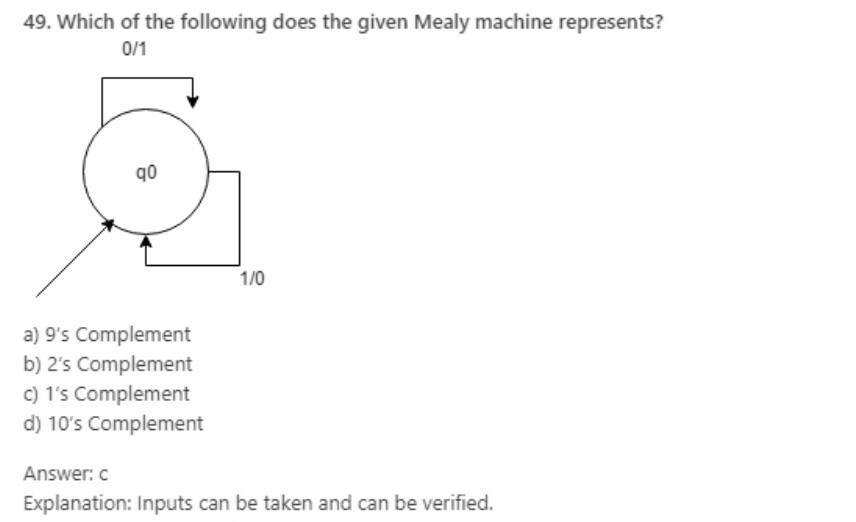50. Which one among the following is true?
A mealy machine
a) produces a language
b) produces a grammar
c) can be converted to NFA
d) has less circuit delays

Explanation: It does not produce a language or a grammar or can be converted to a NFA

Crack Job Placement Aptitude in First Attempt

51. Under which of the following operation, NFA is not closed?
a) Negation
b) Kleene
c) Concatenation
d) None of the mentioned

Explanation: NFA is said to be closed under the following operations:
a) Union
b) Intersection
c) Concatenation
d) Kleene
e) Negation

52. It is less complex to prove the closure properties over regular languages using
a) NFA
b) DFA
c) PDA
d) Can’t be said

Explanation: We use the construction method to prove the validity of closure properties of regular languages. Thus, it can be observe, how tedious and complex is the construction of a DFA as compared to an NFA with respect to space.

53. Which of the following is an application of Finite Automaton?
a) Compiler Design
b) Grammar Parsers
c) Text Search
d) All of the mentioned

Explanation: There are many applications of finite automata, mainly in the field of Compiler Design and Parsers and Search Engines.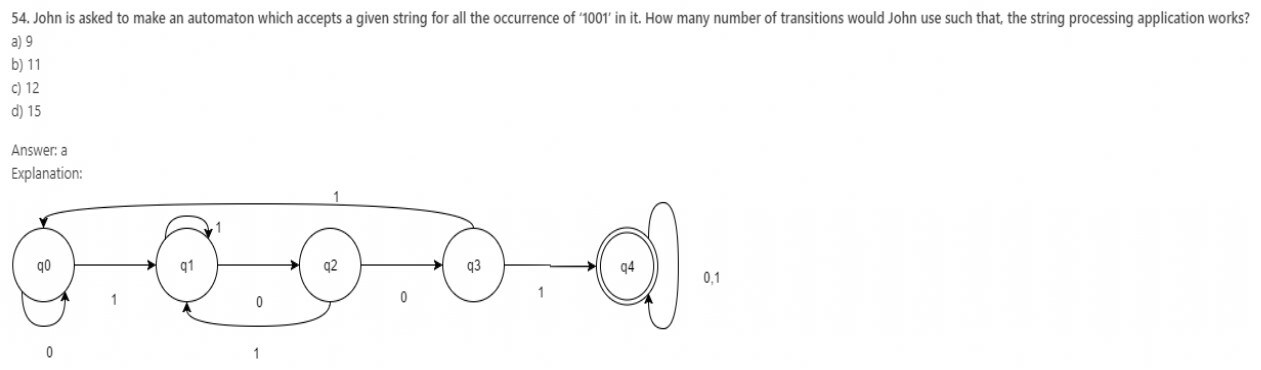55.Which of the following do we use to form an NFA from a regular expression?
a) Subset Construction Method
b) Power Set Construction Method
c) Thompson Construction Method
d) Scott Construction Method

Explanation: Thompson Construction method is used to turn a regular expression in an NFA by fragmenting the given regular expression through the operations performed on the input alphabets.

56. Which among the following can be an example of application of finite state machine(FSM)?
c) Stack
d) None of the mentioned

Explanation: Idle is the state when data in form of packets is send and returns if NAK is received else waits for the NAK to be received.

57. Which among the following is not an application of FSM?
a) Lexical Analyser
b) BOT
c) State charts
d) None of the mentioned

Explanation: Finite state automation is used in Lexical Analyser, Computer BOT (used in games), State charts, etc.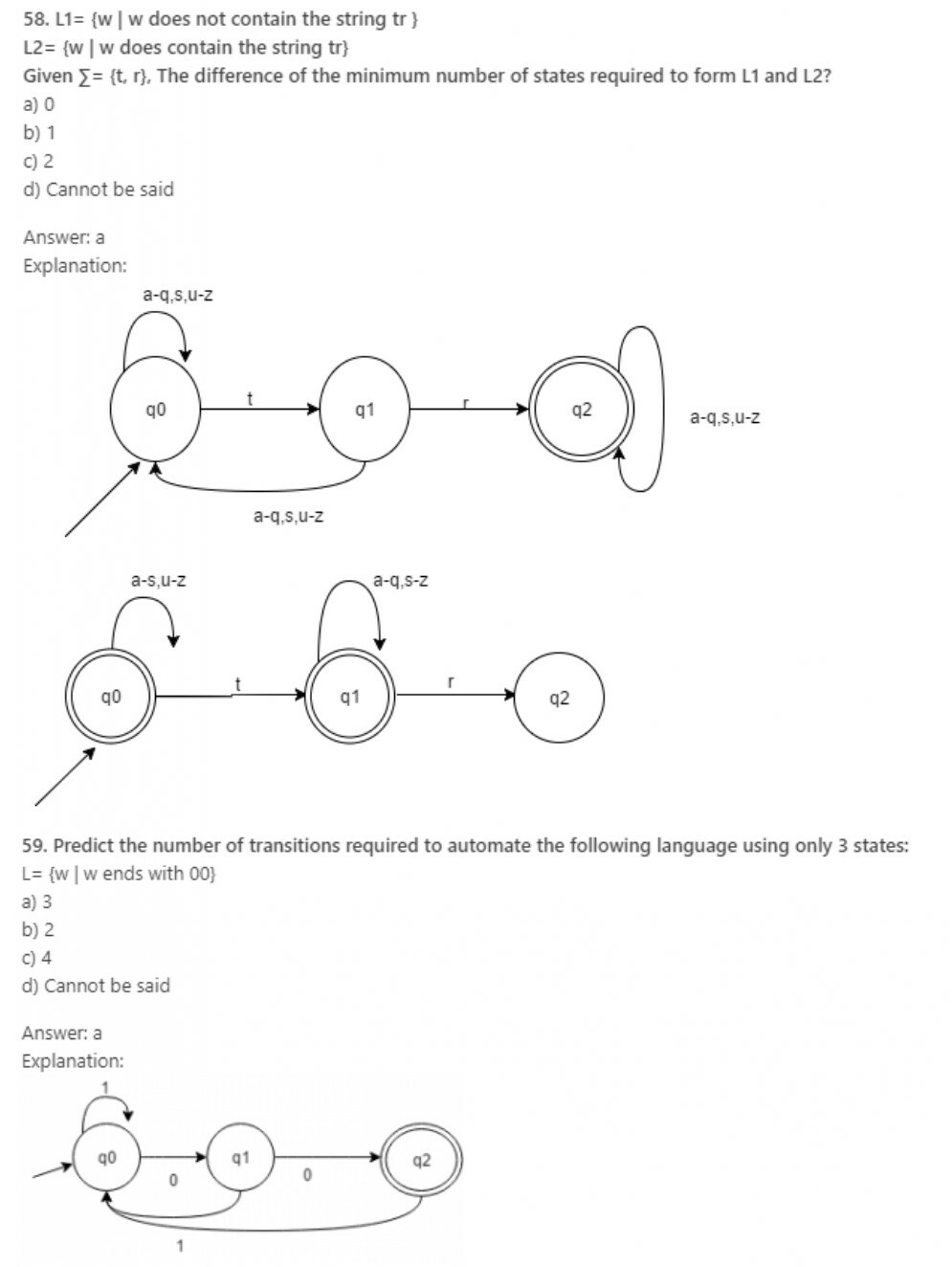60. The total number of states to build the given language using DFA:
L= {w | w has exactly 2 a’s and at least 2 b’s}
a) 10
b) 11
c) 12
d) 13

Explanation: We need to make the number of a as fixed i.e. 2 and b can be 2 or more. Thus, using this condition a finite automata can be created using 1 states.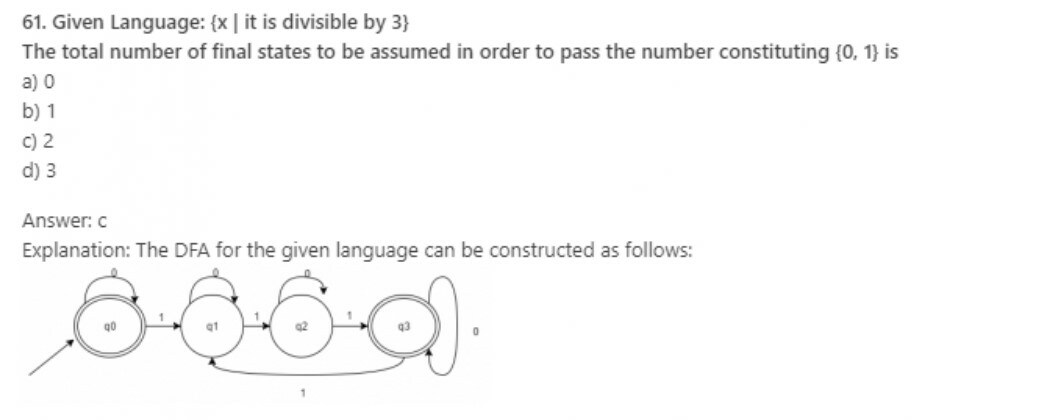Learn Machine Learning with Python from Scratch

62. A binary string is divisible by 4 if and only if it ends with:
a) 100
b) 1000
c) 1100
d) 0011

Explanation: If the string is divisible by four, it surely ends with the substring ‘100’ while a binary string divisible by 2 would surely end with the substring ‘10’.

63. Let L be a language whose FA consist of 5 acceptance states and 11 non final states. It further consists of a dumping state. Predict the number of acceptance states in Lc.
a) 16
b) 11
c) 5
d) 6

Explanation: If L leads to FA1, then for Lc, the FA can be obtained by exchanging the final and non-final states.

64. If L1 and L2 are regular languages, which among the following is an exception?
a) L1 U L2
b) L1 – L2
c) L1 ∩ L2
d) All of the mentioned

Explanation: It the closure property of Regular language which lays down the following statement:
If L1, L2 are 2- regular languages, then L1 U L2, L1 ∩ L2, L1C, L1 – L2 are regular language.

65. Predict the analogous operation for the given language:
A: {[p, q] | p ϵ A1, q does not belong to A2}
a) A1-A2
b) A2-A1
c) A1.A2
d) A1+A2

Explanation: When set operation ‘-‘ is performed between two sets, it points to those values of prior set which belongs to it but not to the latter set analogous to basic subtraction operation.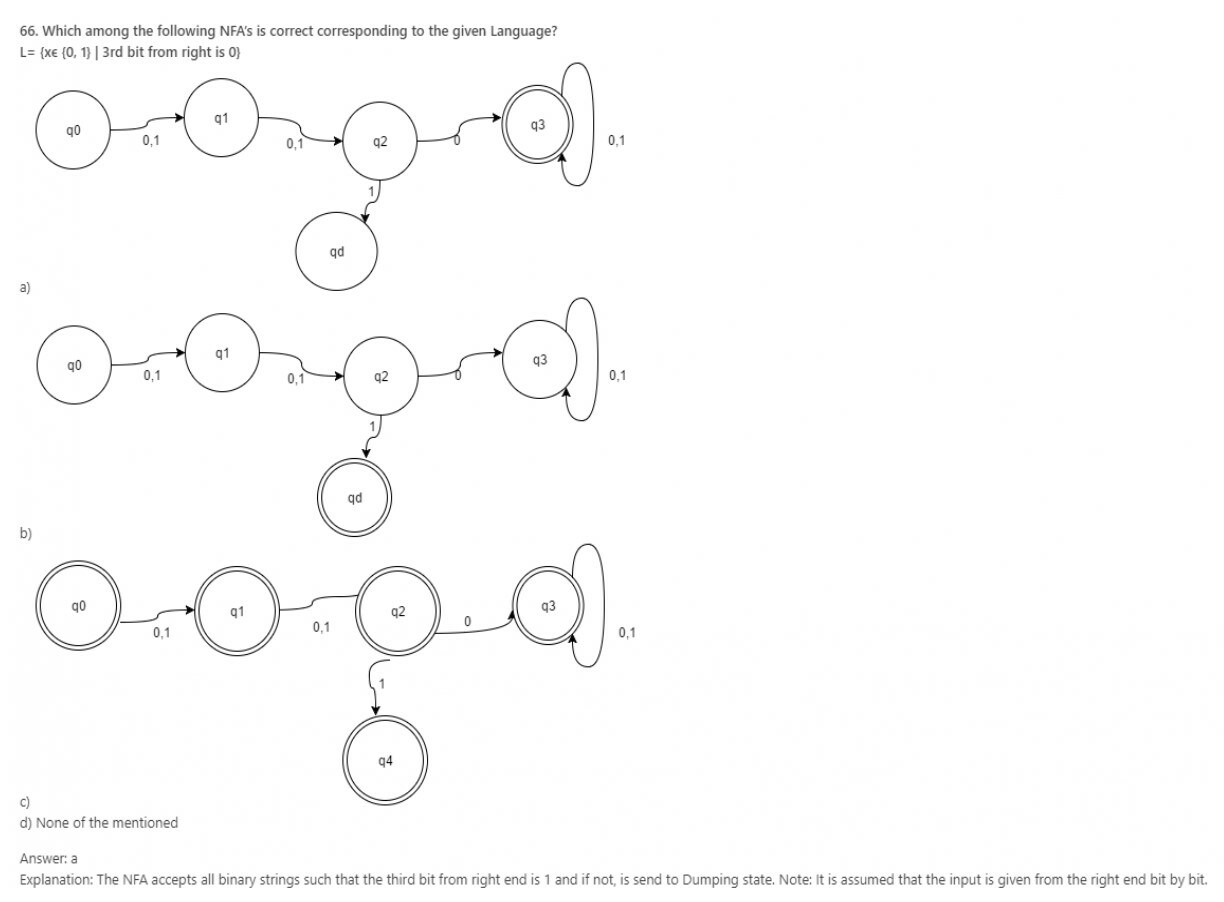67. Statement 1: NFA computes the string along parallel paths.
Statement 2: An input can be accepted at more than one place in an NFA.
Which among the following options are most appropriate?
a) Statement 1 is true while 2 is not
b) Statement 1 is false while is not
c) Statement 1 and 2, both are true
d) Statement 1 and 2, both are false

Explanation: While the machine runs on some input string, if it has the choice to split, it goes in all possible way and each one is different copy of the machine. The machine takes subsequent choice to split further giving rise to more copies of the machine getting each copy run parallel. If any one copy of the machine accepts the strings, then NFA accepts, otherwise it rejects.

68. Which of the following options is correct for the given statement?
Statement: If K is the number of states in NFA, the DFA simulating the same language would have states less than 2k.
a) True
b) False

Explanation: If K is the number of states in NFA, the DFA simulating the same language would have states equal to or less than 2k.

69. Let N (Q, ∑, δ, q0, A) be the NFA recognizing a language L. Then for a DFA (Q’, ∑, δ’, q0’, A’), which among the following is true?
a) Q’ = P(Q)
b) Δ’ = δ’ (R, a) = {q ϵ Q | q ϵ δ (r, a), for some r ϵ R}
c) Q’={q0}
d) All of the mentioned

Explanation: All the optioned mentioned are the instruction formats of how to convert an NFA to a DFA.

70. There exists an initial state, 17 transition states, 7 final states and one dumping state, Predict the maximum number of states in its equivalent DFA?
a) 226
b) 224
c) 225
d) 223
Explanation: The maximum number of states an equivalent DFA can comprise for its respective NFA with k states will be 2k.

Crack Job Placement Aptitude in First Attempt

#### Module 02

1. L is a regular Language if and only If the set of __________ classes of IL is finite.
a) Equivalence
b) Reflexive
c) Myhill
d) Nerode

Explanation: According to Myhill Nerode theorem, the corollary proves the given statement correct for equivalence classes.

2. A language can be generated from simple primitive language in a simple way if and only if
a) It is recognized by a device of infinite states
b) It takes no auxiliary memory
c) Both are correct
d) Both are wrong

Explanation: A language is regular if and only if it can be accepted by a finite automaton. Secondly, It supports no concept of auxiliary memory as it loses the data as soon as the device is shut down.

3. Which of the following does not represents the given language?
Language: {0,01}
a) 0+01
b) {0} U {01}
c) {0} U {0}{1}
d) {0} ^ {01}

Explanation: The given option represents {0, 01} in different forms using set operations and Regular Expressions. The operator like ^, v, etc. are logical operation and they form invalid regular expressions when used.

4. According to the given language, which among the following expressions does it corresponds to?
Language L={xϵ{0,1}|x is of length 4 or less}

a) (0+1+0+1+0+1+0+1)4
b) (0+1)4
c) (01)4
d) (0+1+ε)4

Explanation: The extended notation would be (0+1)4 but however, we may allow some or all the factors to be ε. Thus ε needs to be included in the given regular expression.

5. Which among the following looks similar to the given expression?
((0+1). (0+1)) *
a) {xϵ {0,1} *|x is all binary number with even length}
b) {xϵ {0,1} |x is all binary number with even length}
c) {xϵ {0,1} *|x is all binary number with odd length}
d) {xϵ {0,1} |x is all binary number with odd length}

Explanation: The given regular expression corresponds to a language of binary strings which is of even length including a length of 0.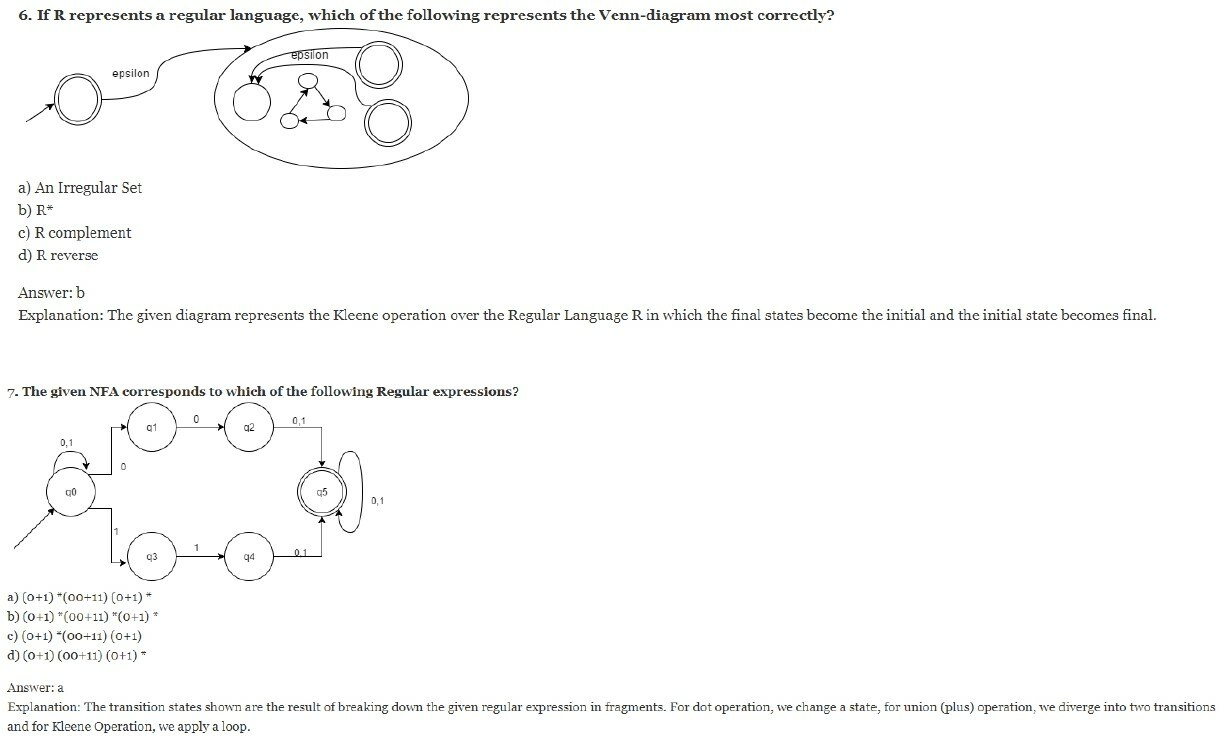Crack Job Placement Aptitude in First Attempt

8. Concatenation Operation refers to which of the following set operations:
a) Union
b) Dot
c) Kleene
d) Two of the options are correct

Explanation: Two operands are said to be performing Concatenation operation AB = A•B = {xy: x ∈ A & y ∈ B}.

9. Concatenation of R with Ф outputs:
a) R
b) Ф
c) R.Ф
d) None of the mentioned

Explanation: By distributive property (Regular expression identities), we can prove the given identity to be Ф.

10. RR* can be expressed in which of the forms:
a) R+
b) R-
c) R+ U R-
d) R

Explanation: RR*=R+ as R+ means the occurrence to be at least once.

Python Programming for Complete Beginners

11. What kind of expressions do we used for pattern matching?
a) Regular Expression
b) Rational Expression
c) Regular & Rational Expression
d) None of the mentioned

Explanation: In automata theory, Regular Expression(sometimes also called the Rational Expression ) is a sequence or set of characters that define a search pattern, mainly for the use in pattern matching with strings or string matching.

12. Which of the following do Regexps do not find their use in?
a) search engines
b) word processors
c) sed
d) none of the mentioned

Explanation: Regexp processors are found in several search engines, seach and replace mechanisms, and text processing utilities.

13. Which of the following languages have built in regexps support?
a) Perl
b) Java
c) Python
d) C++

Explanation: Many languages come with built in support of regexps like Perl, Javascript, Ruby etc. While some provide support using standard libraries like .NET, Java, Python, C++, C and POSIX.

14. The following is/are an approach to process a regexp:
a) Contruction of NFA and subsequently, a DFA.
b) Thompson’s Contruction Algorithm
c) Both (a) and (b)
d) None of the mentioned

Explanation: A regexp processor translates the syntax into internal representation which can be executed and matched with a string and that internal representation can have several approaches like the ones mentioned.

15. Are the given two patterns equivalent?
(1) gray|grey
(2) gr(a|e)y
a) yes
b) no

Explanation: Paranthesis can be used to define the scope and precedence of operators. Thus, both the expression represents the same pattern.

Crack Job Placement Aptitude in First Attempt

16. Which of the following are not quantifiers?
a) Kleene plus +
b) Kleene star *
c) Question mark ?
d) None of the mentioned

Explanation: A quantifier after a token specifies how often the preceding element is allowed to occur. ?, *, +, {n}, {min, }, {min, max} are few quantifiers we use in regexps implementations.

17. Which of the following cannot be used to decide whether and how a given regexp matches a string:
a) NFA to DFA
b) Lazy DFA algorithm
c) Backtracking
d) None of the mentioned

Explanation: There are at least three algorithms which decides for us, whether and how a regexp matches a string which included the transformation of Non deterministic automaton to deterministic finite automaton, The lazy DFA algorithm where one simulates the NFA directly, building each DFA on demand and then discarding it at the next step and the process of backtracking whose running time is exponential.

18. What does the following segment of code output?

```\$string1 = "Hello World\n";
if (\$string1 =~ m/(H..).(l..)/) {
print "We matched '\$1' and '\$2'.\n";
}```

a) We matched ‘Hel’ and ‘ld’
b) We matched ‘Hel’ and ‘lld’
c) We matched ‘Hel’ and ‘lo ‘
d) None of the mentioned

Explanation: () groups a series of pattern element to a single element.
When we use pattern in parenthesis, we can use any of ‘\$1’, ‘\$2’ later to refer to the previously matched pattern.

9. Given segment of code:

```\$string1 = "Hello\nWorld\n";
if (\$string1 =~ m/d\n\z/) {
print "\$string1 is a string ";
print "that ends with 'd\\n'.\n";
}```

What does the symbol /z does?
a) changes line
b) matches the beginning of a string
c) matches the end of a string
d) none of the mentioned

Explanation: It matches the end of a string and not an internal line.The given segment of code outputs:
Hello
World
is a string that ends with ‘d\n’

20. Conversion of a regular expression into its corresponding NFA :
a) Thompson’s Construction Algorithm
b) Powerset Construction
c) Kleene’s algorithm
d) None of the mentioned

Explanation: Thompson construction algorithm is an algorithm in automata theory used to convert a given regular expression into NFA. Similarly, Kleene algorithm is used to convert a finite automaton to a regular expression.

Python Programming for Complete Beginners

21. A regular language over an alphabet a is one that can be obtained from
a) union
b) concatenation
c) kleene
d) All of the mentioned

Explanation: None.

22. Regular expression {0,1} is equivalent to
a) 0 U 1
b) 0 / 1
c) 0 + 1
d) All of the mentioned

Explanation: All are equivalent to union operation.

23. Precedence of regular expression in decreasing order is
a) * , . , +
b) . , * , +
c) . , + , *
d) + , a , *

Explanation: None.

24. Regular expression Φ* is equivalent to
a) ϵ
b) Φ
c) 0
d) 1

Explanation: None.

25. a? is equivalent to
a) a
b) a+Φ
c) a+ϵ
d) wrong expression

Explanation: Zero or one time repetition of previous character .

26. ϵL is equivalent to
a) ϵ
b) Φ
c) L
d) Lϵ

Explanation: None.

27. (a+b)* is equivalent to
a) b*a*
b) (a*b*)*
c) a*b*
d) none of the mentioned

Explanation: None.

28. ΦL is equivalent to
a) LΦ
b) Φ
c) L
d) ϵ

Explanation: None.

29. Which of the following pair of regular expression are not equivalent?
a) 1(01)* and (10)*1
b) x(xx)* and (xx)*x
c) (ab)* and a*b*
d) x+ and x*x+

Explanation: (ab)*=(a*b*)*.

30. Consider following regular expression
i) (a/b)* ii) (a*/b*)* iii) ((ϵ/a)b*)*
Which of the following statements is correct
a) i,ii are equal and ii,iii are not
b) i,ii are equal and i,iii are not
c) ii,iii are equal and i,ii are not
d) all are equal
Explanation: All are equivalent to (a+b)*.

Crack Job Placement Aptitude in First Attempt

31. If L1, L2 are regular and op(L1, L2) is also regular, then L1 and L2 are said to be ____________ under an operation op.
a) open
b) closed
c) decidable
d) none of the mentioned

Explanation: If two regular languages are closed under an operation op, then the resultant of the languages over an operation op will also be regular.

32. Suppose a regular language L is closed under the operation halving, then the result would be:
a) 1/4 L will be regular
b) 1/2 L will be regular
c) 1/8 L will be regular
d) Al of the mentioned

Explanation: At first stage 1/2 L will be regular and subsequently, all the options will be regular.

33. If L1′ and L2′ are regular languages, then L1.L2 will be
a) regular
b) non regular
c) may be regular
d) none of the mentioned

Explanation: Regular language is closed under complement operation. Thus, if L1′ and L2′ are regular so are L1 and L2. And if L1 and L2 are regular so is L1.L2.

34. If L1 and L2′ are regular languages, L1 ∩ (L2′ U L1′)’ will be
a) regular
b) non regular
c) may be regular
d) none of the mentioned

Explanation: If L1 is regular, so is L1′ and if L1′ and L2′ are regular so is L1′ U L2′. Further, regular languages are also closed under intersection operation.

35. If A and B are regular languages, !(A’ U B’) is:
a) regular
b) non regular
c) may be regular
d) none of the mentioned

Explanation: If A and B are regular languages, then A Ç B is a regular language and A ∩ B is equivalent to !(A’ U B’).

Crack Job Placement Aptitude in First Attempt

36. Which among the following are the boolean operations that under which regular languages are closed?
a) Union
b) Intersection
c) Complement
d) All of the mentioned

Explanation: Regular languages are closed under the following operations:
a) Regular expression operations
b) Boolean operations
c) Homomorphism
d) Inverse Homomorphism

37. Suppose a language L1 has 2 states and L2 has 2 states. After using the cross product construction method, we have a machine M that accepts L1 ∩ L2. The total number of states in M:
a) 6
b) 4
c) 2
d) 8

Explanation: M is defined as: (Q, S, d, q0, F)
where Q=Q1*Q2 and F=F1*F2

38. If L is a regular language, then (L’)’ U L will be :
a) L
b) L’
c) f
d) none of the mentioned

Explanation: (L’)’ is equivalent to L and L U L is subsequently equivalent to L.

39. If L is a regular language, then (((L’)r)’)* is:
a) regular
b) non regular
c) may be regular
d) none of the mentioned

Explanation: If L is regular so is its complement, if L’ is regular so is its reverse, if (L’)r is regular so is its Kleene.

40. Which among the following is the closure property of a regular language?
a) Emptiness
b) Universality
c) Membership
d) None of the mentioned
Explanation: All the following mentioned are decidability properties of a regular language. The closure properties of a regular language include union, concatenation, intersection, Kleene, complement , reverse and many more operations.

Python Programming for Complete Beginners

41. Relate the following statement:
Statement: All sufficiently long words in a regular language can have a middle section of words repeated a number of times to produce a new word which also lies within the same language.
a) Turing Machine
b) Pumping Lemma
c) Arden’s theorem
d) None of the mentioned

Explanation: Pumping lemma defines an essential property for every regular language in automata theory. It has certain rules which decide whether a language is regular or not.

42. While applying Pumping lemma over a language, we consider a string w that belong to L and fragment it into _________ parts.
a) 2
b) 5
c) 3
d) 6

Explanation: We select a string w such that w=xyz and |y|>0 and other conditions. However, there exists an integer n such that |w|>=n for any wÎL.

43. If we select a string w such that w∈L, and w=xyz. Which of the following portions cannot be an empty string?
a) x
b) y
c) z
d) all of the mentioned

Explanation: The lemma says, the portion y in xyz cannot be zero or empty i.e. |y|>0, this condition needs to be fulfilled to check the conclusion condition.

44. Let w= xyz and y refers to the middle portion and |y|>0.What do we call the process of repeating y 0 or more times before checking that they still belong to the language L or not?
a) Generating
b) Pumping
c) Producing
d) None of the mentioned

Explanation: The process of repeatation is called pumping and so, pumping is the process we perform before we check whether the pumped string belongs to L or not.

45. There exists a language L. We define a string w such that w∈L and w=xyz and |w| >=n for some constant integer n.What can be the maximum length of the substring xy i.e. |xy|<=?
a) n
b) |y|
c) |x|
d) none of the mentioned

Explanation: It is the first conditional statement of the lemma that states that |xy|<=n, i.e. the maximum length of the substring xy in w can be n only.

Crack Job Placement Aptitude in First Attempt

46. Fill in the blank in terms of p, where p is the maximum string length in L.
Statement: Finite languages trivially satisfy the pumping lemma by having n = ______
a) p*1
b) p+1
c) p-1
d) None of the mentioned

Explanation: Finite languages trivially satisfy the pumping lemma by having n equal to the maximum string length in l plus 1.

47. Answer in accordance to the third and last statement in pumping lemma:
For all _______ xyiz ∈L
a) i>0
b) i<0
c) i<=0
d) i>=0

Explanation: Suppose L is a regular language . Then there is an integer n so that for any x∈L and |x|>=n, there are strings u,v,w so that
x= uvw
|uv|<=n
|v|>0
for any m>=0, uvmw ∈L.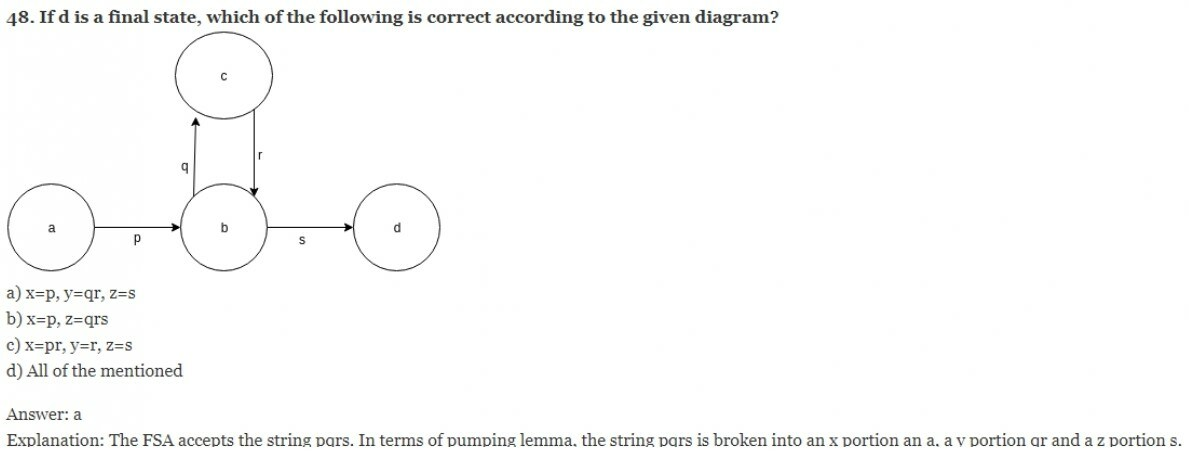49. Let w be a string and fragmented by three variable x, y, and z as per pumping lemma. What does these variables represent?
a) string count
b) string
c) both (a) and (b)
d) none of the mentioned

Explanation: Given: w =xyz. Here, xyz individually represents strings or rather substrings which we compute over conditions to check the regularity of the language.

50. Which of the following one can relate to the given statement:
Statement: If n items are put into m containers, with n>m, then atleast one container must contain more than one item.
a) Pumping lemma
b) Pigeon Hole principle
c) Count principle
d) None of the mentioned

Explanation: Pigeon hole principle states the following example: If there exists n=10 pigeons in m=9 holes, then since 10>9, the pigeonhole principle says that at least one hole has more than one pigeon.

Learn Machine Learning with Python from Scratch

#### Module 03

1. The entity which generate Language is termed as:
a) Automata
b) Tokens
c) Grammar
d) Data
Explanation: The entity which accepts a language is termed as Automata while the one which generates it is called Grammar. Tokens are the smallest individual unit of a program.

2. Production Rule: aAb->agb belongs to which of the following category?
a) Regular Language
b) Context free Language
c) Context Sensitive Language
d) Recursively Ennumerable Language
Explanation: Context Sensitive Language or Type 1 or Linearly Bounded Non deterministic Language has the production rule where the production is context dependent i.e. aAb->agb.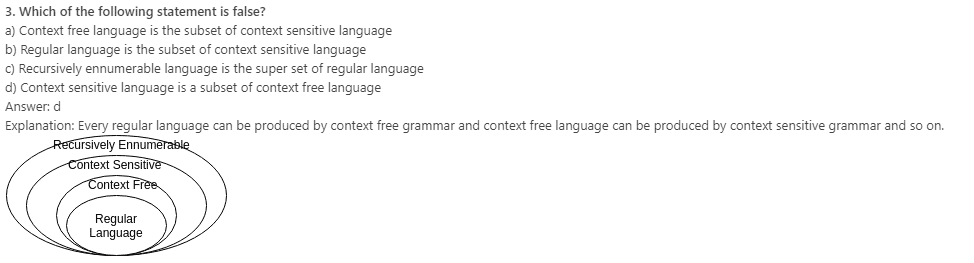4. The Grammar can be defined as: G=(V, ∑, p, S)
In the given definition, what does S represents?
a) Accepting State
b) Starting Variable
c) Sensitive Grammar
d) None of these
Explanation: G=(V, ∑, p, S), here V=Finite set of variables, ∑= set of terminals, p= finite productions, S= Starting Variable.

5. Which among the following cannot be accepted by a regular grammar?
a) L is a set of numbers divisible by 2
b) L is a set of binary complement
c) L is a set of string with odd number of 0
d) L is a set of 0n1n
Explanation: There exists no finite automata to accept the given language i.e. 0n1n. For other options, it is possible to make a dfa or nfa representing the language set.

6. Which of the expression is appropriate?
For production p: a->b where a∈V and b∈_______
a) V
b) S
c) (V+∑)*
d) V+ ∑
Explanation: According to the definition, the starting variable can produce another variable or any terminal or a variable which leads to terminal.

7. For S->0S1|e for ∑={0,1}*, which of the following is wrong for the language produced?
a) Non regular language
b) 0n1n | n>=0
c) 0n1n | n>=1
d) None of the mentioned
Explanation: L={e, 01, 0011, 000111, ……0n1n }. As epsilon is a part of the set, thus all the options are correct implying none of them to be wrong.

8. The minimum number of productions required to produce a language consisting of palindrome strings over ∑={a,b} is
a) 3
b) 7
c) 5
d) 6
Explanation: The grammar which produces a palindrome set can be written as:
S-> aSa | bSb | e | a | b
L={e, a, b, aba, abbbaabbba…..}

9. Which of the following statement is correct?
a) All Regular grammar are context free but not vice versa
b) All context free grammar are regular grammar but not vice versa
c) Regular grammar and context free grammar are the same entity
d) None of the mentioned
Explanation: Regular grammar is a subset of context free grammar and thus all regular grammars are context free.

10. Are ambiguous grammar context free?
a) Yes
b) No
Explanation: A context free grammar G is ambiguous if there is atleast one string in L(G) which has two or more distinct leftmost derivations.

Crack Job Placement Aptitude in First Attempt

11. Which of the following is not a notion of Context free grammars?
a) Recursive Inference
b) Derivations
c) Sentential forms
d) All of the mentioned
Explanation: The following are the notions to express Context free grammars:
a) Recursive Inferences
b) Derivations
c) Sentential form
d) Parse trees

12. State true or false:
Statement: The recursive inference procedure determines that string w is in the language of the variable A, A being the starting variable.
a) true
b) false
Explanation: We apply the productions of CFG to infer that certain strings are in the language of a certain variable.

13. Which of the following is/are the suitable approaches for inferencing?
a) Recursive Inference
b) Derivations
c) Both Recursive Inference and Derivations
d) None of the mentioned
Explanation: Two inference approaches:
1. Recursive inference, using productions from body to head
2. Derivations, using productions from head to body

14. If w belongs to L(G), for some CFG, then w has a parse tree, which defines the syntactic structure of w. w could be:
a) program
b) SQL-query
c) XML document
d) All of the mentioned
Explanation: Parse trees are an alternative representation to derivations and recursive inferences. There can be several parse trees for the same string.

15. Is the following statement correct?
Statement: Recursive inference and derivation are equivalent.
a) Yes
b) No
Explanation: Yes, they are equivalent. Both the terminologies represent the two approaches of recursive inferencing.

16. A->aA| a| b
The number of steps to form aab:
a) 2
b) 3
c) 4
d) 5
Explanation: A->aA=>aaA=>aab

17. An expression is mentioned as follows. Figure out number of incorrect notations or symbols, such that a change in those could make the expression correct.
L(G)={w in T*|S→*w}
a) 0 Errors
b) 1 Error
c) 2 Error
d) Invalid Expression
Explanation: For the given expression, L(G)={w in T*|S→*w}, If G(V, T, P, S) is a CFG, the language of G, denoted by L(G), is the set of terminal strings that have derivations from the start symbol.

18. The language accepted by Push down Automaton:
a) Recursive Language
b) Context free language
c) Linearly Bounded language
d) All of the mentioned
Explanation: Push down automata accepts context free language.

19. Which among the following is the correct option for the given grammar?
G->X111|G1,X->X0|00
a) {0a1b|a=2,b=3}
b) {0a1b|a=1,b=5}
c) {0a1b|a=b}
d) More than one of the mentioned is correct
Explanation: Using the recursive approach, we can conclude that option a is the correct answer, and its not possible for a grammar to have more than one language.

20. Which of the following the given language belongs to?
L={ambmcm| m>=1}
a) Context free language
b) Regular language
c) Both (a) and (b)
d) None of the mentioned
Explanation: The given language is neither accepted by a finite automata or a push down automata. Thus, it is neither a context free language nor a regular language.

Python Programming for Complete Beginners

21. Choose the correct option:
Statement: There exists two inference approaches:
a) Recursive Inference
b) Derivation

a) true
b) partially true
c) false
d) none of the mentioned
Explanation: We apply the productions of a CFG to infer that certain strings are in a language of certain variable.

22. Choose the correct option:
Statement 1: Recursive Inference, using productions from head to body.
Statement 2: Derivations, using productions from body to head.
a) Statement 1 is true and Statement 2 is true
b) Statement 1 and Statement 2, both are false
c) Statement 1 is true and Statement 2 is false
d) Statement 2 is true and Statement 1 is true
Explanation: Both the statements are false. Recursive Inference, using productions from body to head. Derivations, using productions from head to body.

23. Which of the following statements are correct for a concept called inherent ambiguity in CFL?
a) Every CFG for L is ambiguous
b) Every CFG for L is unambiguous
c) Every CFG is also regular
d) None of the mentioned
Explanation: A CFL L is said to be inherently ambiguous if every CFG for L is ambiguous.

24. Which of the theorem defines the existence of Parikhs theorem?
a) Parikh’s theorem
b) Jacobi theorem
c) AF+BG theorem
d) None of the mentioned
Explanation: Rohit Parikh in 1961 proved in his MIT research paper that some context free language can only have ambiguous grammars.

25. State true or false:
Statement: Every right-linear grammar generates a regular language.
a) True
b) False
Explanation: A CFG is said to right linear if each production body has at most one variable, and that variable is at the right end. That is, all productions of a right linear grammar are of the form A->wB or A->w, where A and B are variables while w is some terminal.

26. What the does the given CFG defines?
S->aSbS|bSaS|e and w denotes terminal
a) wwr
b) wSw
c) Equal number of a’s and b’s
d) None of the mentioned
Explanation: Using the derivation approach, we can conclude that the given grammar produces a language with a set of string which have equal number of a’s and b’s.

27. If L1 and L2 are context free languages, which of the following is context free?
a) L1*
b) L2UL1
c) L1.L2
d) All of the mentioned
Explanation: The following is a theorem which states the closure property of context free languages which includes Kleene operation, Union operation and Dot operation.

28. For the given Regular expression, the minimum number of variables including starting variable required to derive its grammar is:
(011+1)*(01)*
a) 4
b) 3
c) 5
d) 6
Explanation: The grammar can be written as:
S->BC
B->AB|ε
A->011|1
C->DC|ε
D->01

29. For the given Regular expression, the minimum number of terminals required to derive its grammar is:
(011+1)*(01)*
a) 4
b) 3
c) 5
d) 6
Explanation: The grammar can be written as:
S->BC
B->AB|ε
A->011|1
C->DC|ε
D->01

30. A grammar G=(V, T, P, S) is __________ if every production taken one of the two forms:
B->aC
B->a

a) Ambiguous
b) Regular
c) Non Regular
d) None of the mentioned
Explanation: The following format of grammar is of Regular grammar and is a part of Context free grammar i.e. like a specific form whose finite automata can be generated.

Crack Job Placement Aptitude in First Attempt

31. Which among the following is a CFG for the given Language:
L={x∈{0,1}*|number of zeroes in x=number of one’s in x}
a) S->e|0S1|1S0|SS
b) S->0B|1A|e A->0S B->1S
c) All of the mentioned

Explanation: We can build context free grammar through different approaches, recursively defining the variables and terminals inorder to fulfil the conditions.

32. Which of the following languages are most suitable for implement context free languages ?
a) C
b) Perl
c) Assembly Language
d) None of the mentioned

Explanation: The advantage of using high level programming language like C and Pascal is that they allow us to write statements that look more like English.

33. Which among the following is the correct grammar for the given language?
L={x∈{0,1}*|number of zeroes in x¹number of one’s in x}
a) S-> 0|SS|1SS|SS1|S1S
b) S-> 1|0S|0SS|SS0|S0S
c) S-> 0|0S|1SS|SS1|S1S
d) None of the mentioned
Explanation: L={0, 1, 00, 11, 001, 010,…}
The grammar can be framed as: S-> 0|0S|1SS|SS1|S1S

34. L={0i1j2k | j>i+k}
Which of the following satisfies the language?
a) 0111100
b) 011100
c) 0001100
d) 0101010
Explanation: It is just required to put the value in the variables in the question and check if it satisfies or not.

35. The most suitable data structure used to represent the derivations in compiler:
a) Queue
c) Tree
d) Hash Tables
Explanation: The tree, known as “Parse tree” when used in a compiler, is the data structure of choice to represent the source program.

36. Which of the following statement is false in context of tree terminology?
a) Root with no children is called a leaf
b) A node can have three children
c) Root has no parent
d) Trees are collection of nodes, with a parent child relationship
Explanation: A node has atmost one parent, drawn above the node, and zero or more children drawn below. Lines connect parents to children. There is one node, one root, that has no parent; this node appears to be at the top of the tree. Nodes with no children are called leaves. Nodes that are not leaves are called interior nodes.

37. In which order are the children of any node ordered?
a) From the left
b) From the right
c) Arbitrarily
d) None of the mentioned
Explanation: The children of a node are ordered from the left and drawn so. If N is to the left of node M, then all the descendents of N are considered to be to the left of all the descendents of M.

38. Which among the following is the root of the parse tree?
a) Production P
b) Terminal T
c) Variable V
d) Starting Variable S
Explanation: The root is labelled by the start symbol. All the leaves are either labelled by a a terminal or with e.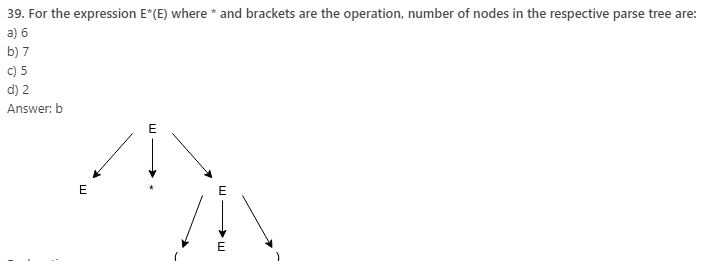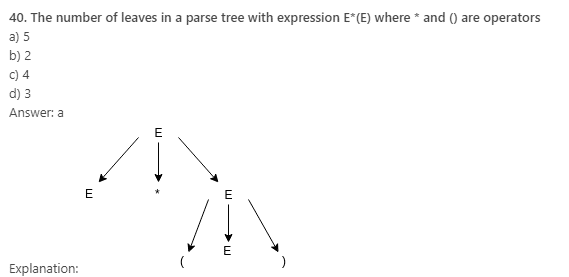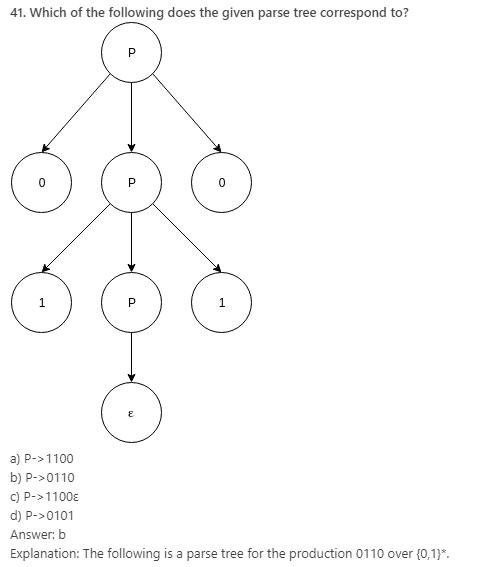42. A grammar with more than one parse tree is called:
a) Unambiguous
b) Ambiguous
c) Regular
d) None of the mentioned
Explanation: A context free grammar G is ambiguous if there is at least one string in L(G) having two or more distinct derivation trees or equivalently, two or more distinct leftmost derivations.

Python Programming for Complete Beginners

43. __________ is the acyclic graphical representation of a grammar.
a) Binary tree
b) Oct tree
c) Parse tree
d) None of the mentioned
Explanation: In order to graphically represent a derivation of a grammar we need to use parse trees.

44. Grammar is checked by which component of compiler
a) Scanner
b) Parser
c) Semantic Analyzer
d) None of the mentioned
Answer: Parser or syntax analyzer is the one responsible for checking the grammar and reporting errors. In this phase, parse tree is generated and syntax is analyzed.

45. To derive a string using the production rules of a given grammar, we use:
a) Scanning
b) Parsing
c) Derivation
d) All of the mentioned
Explanation: Parsing is required to check the acceptability of a string. Further, comes the syntactical phase which is taken care by other phases of compiler.

46. Which of the following parser reaches the root symbol of the tree at last?
a) Top down parser
b) Bottom up parser
c) TOP down and Bottom up parser
d) None of the mentioned
Explanation: Bottom up parser starts from the bottom with the string and comes up to the start symbolusing a parse tree or a derivation tree.

47. Left corner parsing methof uses which of the following?
a) Top down parser
b) Bottom up parser
c) TOP down and Bottom up parser
d) None of the mentioned
Explanation: It is a hybrid method which works bottom up along the left edges of each subtree, and top down on the rest of the parse tree.

48. Which of the following parser performs top down parsing?
a) LALR parser
b) LL parser
c) Recursive Accent parser
d) None of the mentioned
Explanation: Bottom up parsing is done by shift reduce parsers like LALR parsers, Operator precedence parsers, simple precedence parsers, etc.

49. Which of the following is true for shift reduce parsers?
a) Scans and parses the input in one forward pass over the text, without any backup.
b) A shift command advances in the input stream by one symbol
c) LALR parser
d) All of the mentioned
Explanation: The mentioned are the correct and proper functions of a shift reduce parsers. The parsing methods are most commonly used for parsing programming languages, etc.

50. State true or false:
Statement: LALR parsers uses tables rather than mutually recursive functions.
a) true
b) false
Explanation: It is exactly the opposite case where LALR parsers uses mutually recursive functions instead of tables. It is a simplified version of canonical left to right parser.

Crack Job Placement Aptitude in First Attempt

51. LALR in LALR parser stands for:
a) Left aligned left right parser
b) Look ahead left to right parser
c) Language Argument left to right parser
d) None of the mentioned
Explanation: LALR stands for Look ahead left to right parsers. It has more language recognition power than LR(0) parser.

52. Which of the following can be a LALR parser generator?
a) YACC
b) GNU Bison
c) YACC and GNU Bison
d) None of the mentioned
Explanation: YACC is a computer code for UNIX operating system which generates a LALR parser. On the other hand GNU Bison or Bison can generate LALR and GLR parsers.

53. Which of the following parsers do not relate to Bottom up parsing?
a) LL parser
b) Recursive descent parser
c) Earley parsers
d) All of the mentioned
Explanation: All the following mentioned are top down parsers and begin their operation from the starting symbol.

54. Which of the following is true for a predictive parser?
a) Recursive Descent parser
b) no backtracking
c) Recursive Descent parser and no backtracking
d) None of the mentioned
Explanation: Predictive parsing is possible only for the class of LL-grammars, which are the CFG for which there exists some positive integer k that allows a recursive descent parser to decide which production to use by examining only the next k tokens of input.

55. The format: A->aB refers to which of the following?
a) Chomsky Normal Form
b) Greibach Normal Form
c) Backus Naur Form
d) None of the mentioned
Explanation: A context free grammar is in Greibach Normal Form if the right hand sides of all the production rules start with a terminal, optionally followed by some variables.

56. Which of the following does not have left recursions?
a) Chomsky Normal Form
b) Greibach Normal Form
c) Backus Naur Form
d) All of the mentioned
Explanation: The normal form is of the format:
A->aB where the right hand side production tends to begin with a terminal symbo, thus having no left recursions.

57. Every grammar in Chomsky Normal Form is:
a) regular
b) context sensitive
c) context free
d) all of the mentioned
Explanation: Conversely, every context frr grammar can be converted into Chomsky Normal form and to other forms.

58. Which of the production rule can be accepted by Chomsky grammar?
a) A->BC
b) A->a
c) S->e
d) All of the mentioned
Explanation: in CNF, the production rules are of the form:
A->BC
A-> a
S->e

59. Given grammar G:
(1)S->AS
(2)S->AAS
(3)A->SA
(4)A->aa
Which of the following productions denies the format of Chomsky Normal Form?
a) 2,4
b) 1,3
c) 1, 2, 3, 4
d) 2, 3, 4
Explanation: The correct format: A->BC, A->a, X->e.

60. Which of the following grammars are in Chomsky Normal Form:
a) S->AB|BC|CD, A->0, B->1, C->2, D->3
b) S->AB, S->BCA|0|1|2|3
c) S->ABa, A->aab, B->Ac
d) All of the mentioned
Explanation: We can eliminate the options on the basis of the format we are aware of: A->BC, B->b and so on.

Python Programming for Complete Beginners

61. With reference to the process of conversion of a context free grammar to CNF, the number of variables to be introduced for the terminals are:
S->ABa
A->aab
B->Ac
a) 3
b) 4
c) 2
d) 5
Explanation: According to the number of terminals present in the grammar, we need the corresponding that number of terminal variables while conversion.

62. In which of the following, does the CNF conversion find its use?
a) CYK Algorithm
b) Bottom up parsing
c) Preprocessing step in some algorithms
d) All of the mentioned
Explanation: Besides the theoretical significance of CNF, it conversion scheme is helpful in algorithms as a preprocessing step, CYK algorithms and the bottom up parsing of context free grammars.

63. Let G be a grammar. When the production in G satisfy certain restrictions, then G is said to be in ___________.
a) restricted form
b) parsed form
c) normal form
d) all of the mentioned
Explanation: When the production in G satisfy certain restrictions, then G is said to be in ‘normal form’.

64. Let G be a grammar: S->AB|e, A->a, B->b
Is the given grammar in CNF?
a) Yes
b) No
Explanation: e is allowed in CNF only if the starting variable does not occur on the right hand side of the derivation.

65. Which of the following is called Bar-Hillel lemma?
a) Pumping lemma for regular language
b) Pumping lemma for context free languages
c) Pumping lemma for context sensitive languages
d) None of the mentioned
Explanation: In automata theory, the pumping lemma for context free languages, also kmown as the Bar-Hillel lemma, represents a property of all context free languages.

66. Which of the expressions correctly is an requirement of the pumping lemma for the context free languages?
a) uvnwxny
b) uvnwnxny
c) uv2nwx2ny
d) All of the mentioned
Explanation: Let L be a CFL. Then there is an integer n so that for any u that belong to language L satisfying |t| >=n, there are strings u, v, w, x, y and z satisfying
t=uvwxy
|vx|>0
|vwx|<=n For any m>=0, uvnwxny ∈ L

67.Let L be a CFL. Then there is an integer n so that for any u that belong to language L satisfying
|t|>=n, there are strings u, v, w, x, y and z satisfying
t=uvwxy.
Let p be the number of variables in CNF form of the context free grammar. The value of n in terms of p :
a) 2p
b) 2p
c) 2p+1
d) p2
Explanation: This inequation has been derived from derivation tree for t which must have height at least p+2(It has more than 2p leaf nodes, and therefore its height is >p+1).

68. Which of the following gives a positive result to the pumping lemma restrictions and requirements?
a) {aibici|i>=0}
b) {0i1i|i>=0}
c) {ss|s∈{a,b}*}
d) None of the mentioned
Explanation: A positive result to the pumping lemma shows that the language is a CFL and ist contradiction or negative result shows that the given language is not a Context Free language.

69. Using pumping lemma, which of the following cannot be proved as ‘not a CFL’?
a) {aibici|i>=0}
b) {ss|s∈{a,b}*}
c) The set legal C programs
d) None of the mentioned
Explanation: There are few rules in C that are context dependent. For example, declaration of a variable before it can be used.

70. State true or false:
Statement: We cannot use Ogden’s lemma when pumping lemma fails.
a) true
b) false
Explanation: Although the pumping lemma provides some information about v and x that are pumped, it says little about the location of these substrings in the string t. It can be used whenever the pumping lemma fails. Example: {apbqcrds|p=0 or q=r=s}, etc.

Learn Machine Learning with Python from Scratch

71. Which of the following cannot be filled in the blank below?
Statement: There are CFLs L1 nad L2 so that ___________is not a CFL.
a) L1∩L2
b) L1′
c) L1*
d) None of the mentioned
Explanation: A set of context free language is closed under the following operations:
a) Union
b) Concatenation
c) Kleene

72. The pumping lemma is often used to prove that a language is:
a) Context free
b) Not context free
c) Regular
d) None of the mentioned
Explanation: The pumping lemma is often used to prove that a given language L is non-context-free, by showing that arbitrarily long strings s are in L that cannot be “pumped” without producing strings outside L.

73. What is the pumping length of string of length x?
a) x+1
b) x
c) x-1
d) x2
Explanation: There exists a property of all strings in the language that are of length p, where p is the constant-called the pumping length .For a finite language L, p is equal to the maximum string length in L plus 1.

74. Which of the following does not obey pumping lemma for context free languages ?
a) Finite languages
b) Context free languages
c) Unrestricted languages
d) None of the mentioned
Explanation: Finite languages (which are regular hence context-free ) obey pumping lemma whereas unrestricted languages like recursive languages do not obey pumping lemma for context-free languages.

Crack Job Placement Aptitude in First Attempt

#### Module 04

1. The production of the form A->B , where A and B are non terminals is called
a) Null production
b) Unit production
c) Greibach Normal Form
d) Chomsky Normal Form
Explanation: A->ε is termed as Null production while A->B is termed as Unit production.

2. Halting states are of two types. They are:
a) Accept and Reject
b) Reject and Allow
c) Start and Reject
d) None of the mentioned
Explanation: Halting states are the new tuple members introduced in turing machine and is of two types: Accept Halting State and Reject Halting State.

3. A push down automata can be represented as:
PDA= ε-NFA +[stack] State true or false:
a) true
b) false
Explanation: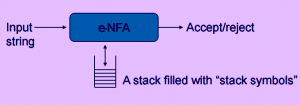4. A pushdown automata can be defined as: (Q, ∑, G, q0, z0, A, d)
What does the symbol z0 represents?
a) an element of G
b) initial stack symbol
c) top stack alphabet
d) all of the mentioned
Explanation: z0 is the initial stack symbol, is an element of G. Other symbols like d represents the transition function of the machine.

5. Which of the following correctly recognize the symbol ‘|-‘ in context to PDA?
a) Moves
b) transition function
c) or/not symbol
d) none of the mentioned
Explanation: Using this notation, we can define moves and further acceptance of a string by the machine.

Crack Job Placement Aptitude in First Attempt

6. Which among the following is true for the given statement?
Statement :If there are strings R and T in a language L so that R is prefix of T and R is not equivalent to T.
a) No DPDA can accept L by empty stack
b) DPDA can accept L by an empty stack
c) L is regular
d) None of the mentioned
Explanation: If M is a DPDA accepting L by an empty stsck, R and T are distinct strings in L, and R is a prefix of T, then the sequence of moves M must make in order to accept R leaves the stack empty, since R∈L. But then T cannot be accepted, since M cant move with an empty stack.

7. Which of the following can be accepted by a DPDA?
a) The set of even length palindrome over {a,b}
b) The set of odd length palindrome over {a,b}
c) {xxc| where c stands for the complement,{0,1}}
d) None of the mentioned
Explanation: Theorem: The language pal of palindromes over the alphabet {0,1} cannot be accepted by any finite automaton , and it is therefore not regular.

8. For a counter automaton, with the symbols A and Z0, the string on the stack is always in the form of __________
a) A
b) AnZ0, n>=0
c) Z0An, n>=0
d) None of the mentioned
Explanation:The possible change in the stack contents is a change in the number of A’s on the stack.

9. State true or false:
Statement: Counter Automaton can exist for the language L={0i1i|i>=0}
a) true
b) false
Explanation: The PDA works as follows. Instead of saving excess 0’s or 1’s on the stack, we save *’s and use two different states to indicate which symbol there is currently a surplus of. The state q0 is the initial state and the only accepting state.

10. Let ∑={0,1}* and the grammar G be:
S->ε
S->SS
S->0S1|1S0
State which of the following is true for the given
a) Language of all and only Balanced strings
b) It contains equal number of 0’s and 1’s
c) Ambiguous Grammar
d) All of the mentioned
Explanation: A string is said to be balanced if it consist of equal number of 0’s and 1’s.

Python Programming for Complete Beginners

11. A push down automaton employs ________ data structure.
a) Queue
c) Hash Table
d) Stack
Explanation: A push down automata uses a stack to carry out its operations. They are more capable than the finite automatons but less than the turing model.

12. State true or false:
Statement: The operations of PDA never work on elements, other than the top.
a) true
b) false
Explanation: The term pushdown refers to the fact that the elements are pushed down in the stack and as per the LIFO principle, the operation is always performed on the top element of the stack.

13. Which of the following allows stacked values to be sub-stacks rather than just finite symbols?
a) Push Down Automaton
b) Turing Machine
c) Nested Stack Automaton
d) None of the mentioned
Explanation: In computational theory, a nested stack automaton is a finite automaton which makes use of stack containing data which can be additional stacks.

14. A non deterministic two way, nested stack automaton has n-tuple definition. State the value of n.
a) 5
b) 8
c) 4
d) 10
Explanation: The 10-tuple can be stated as: NSA= ‹Q,Σ,Γ,δ,q0,Z0,F,[,],]›.

15. Push down automata accepts _________ languages.
a) Type 3
b) Type 2
c) Type 1
d) Type 0
Explanation: Push down automata is for Context free languages and they are termed as Type 2 languages according to Chomsky hierarchy.

16. The class of languages not accepted by non deterministic, nonerasing stack automata is _______
a) NSPACE(n2)
b) NL
c) CSL
d) All of the mentioned
Explanation: NSPACE or non deterministic space is the computational resource describing the memory space for a non deterministic turing machine.

17. A push down automaton with only symbol allowed on the stack along with fixed symbol.
a) Embedded PDA
b) Nested Stack automata
c) DPDA
d) Counter Automaton
Explanation: This class of automata can recognize a set of context free languages like {anbn|n belongs to N}

18. Which of the operations are eligible in PDA?
a) Push
b) Delete
c) Insert
d) Pop
Explanation: Push and pop are the operations we perform to operate a stack. A stack follows the LIFO principle, which states its rule as: Last In First Out.

19. A string is accepted by a PDA when
a) Stack is empty
b) Acceptance state
c) Both (a) and (b)
d) None of the mentioned
Explanation: When we reach the acceptance state and find the stack to be empty, we say, the string has been accepted by the push down automata.

20. The following move of a PDA is on the basis of:
a) Present state
b) Input Symbol
c) Both (a) and (b)
d) None of the mentioned
Explanation: The next operation is performed by PDA considering three factors: present state,symbol on the top of the stack and the input symbol.

Crack Job Placement Aptitude in First Attempt

21. If two sets, R and T has no elements in common i.e. RÇT=Æ, then the sets are called
a) Complement
b) Union
c) Disjoint
d) Connected
Explanation: Two sets are called disjoint if they have no elements in common i.e. RÇT=Æ.

22. Which among the following is not a part of the Context free grammar tuple?
a) End symbol
b) Start symbol
c) Variable
d) Production
Explanation: The tuple definition of context free grammar is: (V, T, P, S) where V=set of variables, T=set of terminals, P=production, S= Starting Variable.

23. A context free grammar is a ___________
a) English grammar
b) Regular grammar
c) Context sensitive grammar
d) None of the mentioned
Explanation: Context free grammar is the set which belongs to the set of context free grammar. Similarly, Regular grammar is a set which belongs to the the set of Context free grammar.

24. The closure property of context free grammar includes :
a) Kleene
b) Concatenation
c) Union
d) All of the mentioned
Explanation: Context free grammars are closed under kleene operation, union and concatenation too.

25. Which of the following automata takes stack as auxiliary storage?
a) Finite automata
b) Push down automata
c) Turing machine
d) All of the mentioned
Explanation: Pushdown Automaton uses stack as an auxiliary storage for its operations. Turing machines use Queue for the same.

26. Which of the following automata takes queue as an auxiliary storage?
a) Finite automata
b) Push down automata
c) Turing machine
d) All of the mentioned
Explanation: Pushdown Automaton uses stack as an auxiliary storage for its operations. Turing machines use Queue for the same.

27. A context free grammar can be recognized by
a) Push down automata
b) 2 way linearly bounded automata
c) Both (a) and (b)
d) None of the mentioned
Explanation: A linearly bounded automata is a restricted non deterministic turing machine which is capable of accepting ant context free grammar.

28. A null production can be referred to as:
a) String
b) Symbol
c) Word
d) All of the mentioned
Explanation: Null production is always taken as a string in computational theory.

29. The context free grammar which generates a Regular Language is termed as:
a) Context Regular Grammar
b) Regular Grammar
c) Context Sensitive Grammar
d) None of the mentioned
Explanation: Regular grammar is a subset of Context free grammar. The CFGs which produces a language for which a finite automaton can be created is called Regular grammar.

30. NPDA stands for
a) Non-Deterministic Push Down Automata
b) Null-Push Down Automata
c) Nested Push Down Automata
d) All of the mentioned
Explanation: NPDA stands for non-deterministic push down automata whereas DPDA stands for deterministic push down automata.

Python Programming for Complete Beginners

31. The transition a Push down automaton makes is additionally dependent upon the:
a) stack
b) input tape
c) terminals
d) none of the mentioned
Explanation: A PDA is a finite machine which has an additional stack storage. Its transitions are based not only on input and the correct state but also on the stack.

32. A PDA machine configuration (p, w, y) can be correctly represented as:
a) (current state, unprocessed input, stack content)
b) (unprocessed input, stack content, current state)
c) (current state, stack content, unprocessed input)
d) none of the mentioned
Explanation: A machine configuration is an element of K×Σ*×Γ*.
(p,w,γ) = (current state, unprocessed input, stack content).

33. |-* is the __________ closure of |-
a) symmetric and reflexive
b) transitive and reflexive
c) symmetric and transitive
d) none of the mentioned
Explanation: A string w is accepted by a PDA if and only if (s,w, e) |-* (f, e, e)

34. With reference of a DPDA, which among the following do we perform from the start state with an empty stack?
a) process the whole string
b) end in final state
c) end with an empty stack
d) all of the mentioned
Explanation: The empty stack in the end is our requirement relative to finite state automatons.

35. A DPDA is a PDA in which:
a) No state p has two outgoing transitions
b) More than one state can have two or more outgoing transitions
c) Atleast one state has more than one transitions
d) None of the mentioned
Explanation: A Deterministic Push Down Automata is a Push Down Automata in which no state p has two or more transitions.

36. State true or false:
Statement: For every CFL, G, there exists a PDA M such that L(G) = L(M) and vice versa.
a) true
b) false
Explanation: There exists two lemma’s such that:
a) Given a grammar G, construct the PDA and show the equivalence
b) Given a PDA, construct a grammar and show the equivalence

37. If the PDA does not stop on an accepting state and the stack is not empty, the string is:
a) rejected
b) goes into loop forever
c) both (a) and (b)
d) none of the mentioned
Explanation: To accept a string, PDA needs to halt at an accepting state and with a stack empty, else it is called rejected. Given a PDA M, we can construct a PDA M’ that accepts the same language as M, by both acceptance criteria.

38. A language accepted by Deterministic Push down automata is closed under which of the following?
a) Complement
b) Union
c) Both (a) and (b)
d) None of the mentioned
Explanation: Deterministic Context free languages(one accepted by PDA by final state), are drastically different from the context free languages. For example they are closed under complementation and not union.

39. Which of the following is a simulator for non deterministic automata?
a) JFLAP
b) Gedit
c) FAUTO
d) None of the mentioned
Explanation: JFLAP is a software for experimenting with formal topics including NFA, NPDA, multi-tape turing machines and L-systems.

40. Finite-state acceptors for the nested words can be:
a) nested word automata
b) push down automata
c) ndfa
d) none of the mentioned
Explanation: The linear encodings of languages accepted by finite nested word automata gives the class of ‘visibly pushdown automata’.

Learn Machine Learning with Python from Scratch

41. Which of the following is analogous to the following?
:NFA and NPDA
a) Regular language and Context Free language
b) Regular language and Context Sensitive language
c) Context free language and Context Sensitive language
d) None of the mentioned
Explanation: All regular languages can be accepted by a non deterministic finite automata and all context free languages can be accepted by a non deterministic push down automata.

42. Let T={p, q, r, s, t}. The number of strings in S* of length 4 such that no symbols can be repeated.
a) 120
b) 625
c) 360
d) 36
Explanation: Using the permutation rule, we can calculate that there will be total of 625 permutations on 5 elements taking 4 as the length.

43. Which of the following relates to Chomsky hierarchy?
a) Regular<CFL<CSL<Unrestricted
b) CFL<CSL<Unrestricted<Regular
c) CSL<Unrestricted<CF<Regular
d) None of the mentioned
Explanation: The chomsky hierarchy lays down the following order: Regular<CFL<CSL<Unrestricted

44. A language is accepted by a push down automata if it is:
a) regular
b) context free
c) both (a) and (b)
d) none of the mentioned
Explanation: All the regular languages are the subset to context free languages and thus can be accepted using push down automata.

45. Which of the following is an incorrect regular expression identity?
a) R+f=R
b) eR=e
c) Rf=f
d) None of the mentioned
Explanation: e is the identity for concatenation. Thus, eR=R.

46. Which of the following strings do not belong the given regular expression?
(a)*(a+cba)
a) aa
b) aaa
c) acba
d) acbacba
Explanation: The string acbacba is unacceptable by the regular expression (a)*(a+cba).

47. Which of the following regular expression allows strings on {a,b}* with length n where n is a multiple of 4.
a) (a+b+ab+ba+aa+bb+aba+bab+abab+baba)*
b) (bbbb+aaaa)*
c) ((a+b)(a+b)(a+b)(a+b))*
d) None of the mentioned
Explanation: Other mentioned options do not many of the combinations while option c seems most reliable.

48. Which of the following strings is not generated by the given grammar:
S->SaSbS|e
a) aabb
b) abab
c) abaabb
d) None of the mentioned
Explanation: All the given options are generated by the given grammar. Using the methods of left and right derivations, it is simpler to look for string which a grammar can generate.

49. abb*c denotes which of the following?
a) {abnc|n=0}
b) {abnc|n=1}
c) {anbc|n=0}
d) {abcn|n>0}